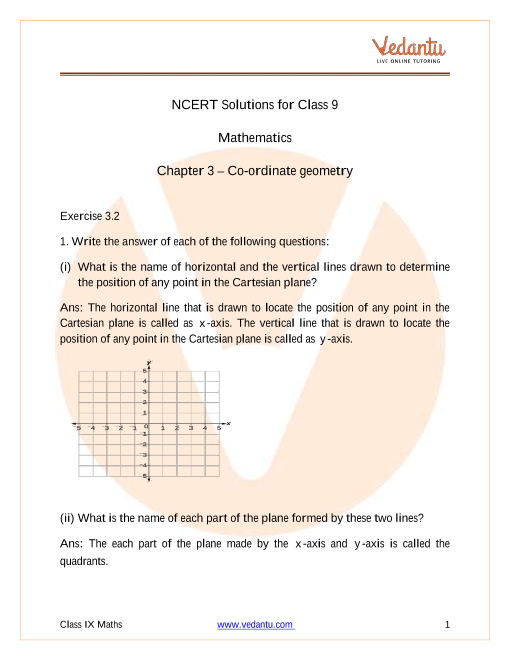# NCERT Solutions for Class 9 Maths Chapter 3 Coordinate Geometry (Ex 3.2) Exercise 3.2

## NCERT Solutions for Class 9 Maths Chapter 3 Exercise 3.2 (Ex 3.2)Free PDF download of NCERT Solutions for Class 9 Maths Chapter 3 Exercise 3.2 (Ex 3.2) and all chapter exercises at one place prepared by an expert teacher as per NCERT (CBSE) books guidelines. Class 9 Maths Chapter 3 Coordinate Geometry Exercise 3.2 Questions with Solutions to help you to revise the complete Syllabus and Score More marks. Register and get all NCERT Book solutions in your emails. You can also download NCERT Solutions for Class 9 Maths to help you to revise the complete syllabus and score more marks in your examinations. Subjects like Science, Maths, English will become easy to study if you have access to NCERT Solution for Class 9 Science, Maths solutions, and solutions of other subjects that are available on Vedantu only.

Do you need help with your Homework? Are you preparing for Exams?
Study without Internet (Offline)## Access NCERT Solutions for Class 9 Maths Chapter 3 – Coordinate Geometry

### Exercise 3.2

#### 1. Write the answer of each of the following questions:

(i). What is the name of horizontal and the vertical lines drawn to determine the position of any point in the Cartesian plane?

(ii). What is the name of each part of the plane formed by these two lines?

(iii). Write the name of the point where these two lines intersect.

Ans. (i) The horizontal line that is drawn to locate the position of any point in the Cartesian plane is called the $x$-axis.

The vertical line that is drawn to locate the position of any point in the Cartesian plane is called the $y$-axis.

(ii) Each part of the plane made by the $x$-axis and $y$-axis is called the quadrants.

(iii) The point where the $x$-axis and the $y$-axis meets is called as the origin ($O$).

#### 2. See the figure, and write the following:

1. The coordinates of $\mathbf{B}$.

2. The coordinates of $\mathbf{C}$.

3. The point identified by the coordinates $\left( \mathbf{-3,-5} \right)$.

4. The point identified by the coordinates $\left( \mathbf{2,-4} \right)$.

5. The abscissa of the point $\mathbf{D}$.

6. The ordinate of the point $\mathbf{H}$.

7. The coordinates of the point $\mathbf{L}$.

8. The coordinates of the point $\mathbf{M}$.

Ans. Following the figure given in the problem, we can conclude that:

1. The coordinates of point $B$ is the distance of point $B$ from $x$-axis and $y-$axis. So, the coordinates of point $B$ are $\left( -5,2 \right)$.

2. The coordinates of point $C$ is the distance of point $C$ from $x$-axis and $y$-axis. So, the coordinates of point $C$ are $\left( 5,-5 \right)$.

3. The point that represents the coordinates $\left( -3,-5 \right)$ is $E$.

4. The point that represents the coordinates $\left( 2,-4 \right)$ is $G$.

5. The abscissa of point $D$ is the distance of point $D$ from the $y$-axis. So, the abscissa of point $D$ is $6$.

6. The ordinate of point $H$ is the distance of point $H$ from the $x$-axis. Therefore, the abscissa of point $H$ is $-3$.

7. The coordinates of point $L$ in the above figure is the distance of point $L$ from $x$-axis and $y$-axis. So, the coordinates of point $L$ are $\left( 0,5 \right)$.

8. The coordinates of point $M$ in the above figure is the distance of point $M$ from $x$-axis and $y$-axis. So, the coordinates of point $M$ are $\left( -3,0 \right)$.

## NCERT Solutions for Class 9 Maths Chapter 3 Coordinate Geometry Exercise 3.2

Opting for the NCERT solutions for Ex 3.2 Class 9 Maths is considered as the best option for the CBSE students when it comes to exam preparation. This chapter consists of many exercises. Out of which we have provided the Exercise 3.2 Class 9 Maths NCERT solutions on this page in PDF format. You can download this solution as per your convenience or you can study it directly from our website/ app online.

Vedantu in-house subject matter experts have solved the problems/ questions from the exercise with the utmost care and by following all the guidelines by CBSE. Class 9 students who are thorough with all the concepts from the Subject Coordinate Geometry textbook and quite well-versed with all the problems from the exercises given in it, then any student can easily score the highest possible marks in the final exam. With the help of this Class 9 Maths Chapter 3 Exercise 3.2 solutions, students can easily understand the pattern of questions that can be asked in the exam from this chapter and also learn the marks weightage of the chapter. So that they can prepare themselves accordingly for the final exam.

Besides these NCERT solutions for Class 9 Maths Chapter 3 Exercise 3.2, there are plenty of exercises in this chapter which contain innumerable questions as well. All these questions are solved/answered by our in-house subject experts as mentioned earlier. Hence all of these are bound to be of superior quality and anyone can refer to these during the time of exam preparation. In order to score the best possible marks in the class, it is really important to understand all the concepts of the textbooks and solve the problems from the exercises given next to it.

Do not delay any more. Download the NCERT solutions for Class 9 Maths Chapter 3 Exercise 3.2 from Vedantu website now for better exam preparation. If you have the Vedantu app in your phone, you can download the same through the app as well. The best part of these solutions is these can be accessed both online and offline as well.SHARETWEETSHARESUBSCRIBE